Science Journal of Applied Mathematics and Statistics
Volume 4, Issue 3, June 2016, Pages: 88-96

Comparative Study of Backpropagation Algorithms in Forecasting Volatility of Crude Oil Price in Nigeria

S. Suleiman1, S. U. Gulumbe1, B. K. Asare1, M. Abubakar2

1Department of Mathematics, Usmanu Danfodiyo University, Sokoto, Nigeria

2Department of Economics, Usmanu Danfodiyo University, Sokoto, Nigeria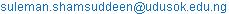(S. Suleiman)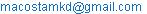(S. Suleiman)

S. Suleiman, S. U. Gulumbe, B. K. Asare, M. Abubakar. Comparative Study of Backpropagation Algorithms in Forecasting Volatility of Crude Oil Price in Nigeria. Science Journal of Applied Mathematics and Statistics. Vol. 4, No. 3, 2016, pp. 88-96. doi: 10.11648/j.sjams.20160403.11

Received: April 5, 2016; Accepted: April 19, 2016; Published: May 7, 2016

Abstract: This paper explores the application of artificial neural network in volatility forecasting. A recurrent neural network has been integrated in to GARCH model to form the hybrid model called GARCH-Neural model. The emphasis of the research is to investigate the performance of the variants of Backpropagation algorithms in training the proposed GARCH-neural model. In the first place, EGARCH (3, 3) was identified in this paper most preferred model describing crude oil price volatility in Nigeria. Similarly, Levenberg-Marquardt (LM) training algorithms were found to be fastest in convergence and also provide most accurate predictions of the volatility when to other training techniques.

Keywords: GARH Models, Recurrent Neural Networks, Backpropagation Algorithms and Forecasting

Contents

1. Introduction

Crude oil is considered to be an important export commodity in Nigeria because of its contribution to the economy of the country. It was first discovered in 1958 and first produced well in 1956. Before that time, the country mainly depends on the exports of the agricultural commodities that comprised groundnuts, cocoa beans, palm oil, cotton and rubber. Palm oil was the leading export from 1946-1958, followed by cocoa beans while groundnut/oil ranked third. From a production level of 1.9 million barrels per day in 1958, crude oil exports rose to 2.35 million barrels per day in the early 2000s. However, it had fluctuated between 2.35 and 2.40 million barrels per day between 2011 and 2015 which was far below the OPEC quota due to the socio-political instability in the oil-producing areas of the country. In terms of its contribution to total revenue, receipts from oil that constituted 26.3 per cent of the federally collected-revenue in 1970, rose to 82.1 per cent in 1974 and 83.0 per cent in 2008 largely on account of a rise in crude oil prices at the international market.

Over the last two years, global oil prices have been dropping and bearing in mind that Nigeria is an import dependent economy, this development is worrisome. Our reviews of the current oil exports also reveal a southward trend due to significant oil theft and lower global demands. Indeed, NNPC (Nigerian National Petroleum Corporation) puts total value of revenue loss due to oil theft at \$11bn in 2013.

More importantly, crude oil for the last three decades has been the major source of revenue, energy and the foreign exchange for the Nigerian economy. In 2000 oil and gas export earnings accounted for about 98% and about 83% of federal government revenue. .

The term volatility has been given different definitions by different scholars across disciplines. In relation to crude oil price, volatility is the variation in the worth of a variable, especially price . Volatility is the measure of the tendency of oil price to rise or fall sharply within a period of time, such as a day, a month or a year .  Defines volatility as the standard deviation in a given period. She notes that volatility has a negative and significant impact on economic growth instantly, while the impact of oil price changes delays until after a year. She concludes by saying that ―it is volatility/change in crude oil prices rather than oil price level that has a significant influence on economic growth. In a nutshell, volatility is a measurement of the fluctuations (i.e rise and fall) of the price of commodity for example oil price over a period of time.

Artificial neural networks (ANNs) have been known to have the capability to learn the complex approximate relationships between the inputs and the outputs of the system and are not restricted by the size and complexity of the system . The ANNs learn these approximate relationships on the basis of actual inputs and outputs. Therefore, they are generally more accurate as compared to the relationships based on assumptions. ANNs have been used for volatility forecasting in several papers for example [6-10]. Despite their popularity in applications to financial variables, ANNs have not been utilized very well in Nigerian financial market. Similarly, multi-layer feedforward Artificial Neural Networks using Backpropagation algorithms for training have been used in several literatures for example [11-14]. Since the Backpropagation algorithm has been successfully applied to train neural networks, this work aims to investigate the training performance of the some variants of the back propagation algorithm in training the proposed model for forecasting volatility.

2. Volatility Models

Letbe stock price at time. Then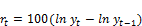(1)

denotes the continuously compounded daily returns of the underlying assets at time.

The most widely used model for estimating volatility is ARCH (Auto Regressive Conditional Heteroscedasticity) model developed by  as also contained in . Since the development of the original ARCH model, a lot of research has been carried out on extensions of this model among which GARCH  and is defined as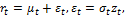and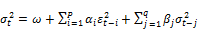(2)are non-negative parameters to be estimated,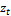is an independently and identically distributed (i.i.d.) random variables with zero mean and unit variance and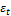is a serially uncorrelated sequence with zero mean and the conditional variance of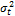which may be nonstationary, the GARCH model reduces the number of parameters necessary when information in the lag (s) of the conditional variance in addition to the lagged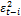terms were considered, but was not able to account for asymmetric behavior of the returns.

Because of this weakness of GARCH model, a number of extensions of the GARCH (p, q) model have been developed to explicitly account for the skewness or asymmetry. The popular models of asymmetric volatility includes, the exponential GARCH (EGARCH) model,  GJR-GARCH model, asymmetric power ARCH (APARCH).

The GJR-GARCH (p, q) model was introduced by  to allow for allows asymmetric effects. The model is given as: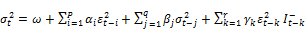(3)

Where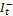(a dummy variable) =1 if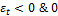otherwise.

In the GJR-GARCH model, good news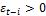and bad news,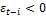, have differential effects on the conditional variance; good news has an impact of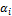while bad news has an impact of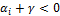. If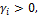bad news increases volatility, and there is a leverage effect for the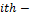order. if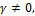the news impact is asymmetric . The exponential GARCH (EGARCH) model advanced by  is the earliest extension of the GARCH model that incorporates asymmetric effects in returns from speculative prices. The EGARCH model is defined as follows: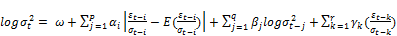(4)

Where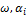,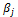and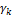are constant parameters. The EGARCH (p, q) model, unlike the GARCH (p, q) model, indicates that the conditional variance is an exponential function, thereby removing the need for restrictions on the parameters to ensure positive conditional variance. The asymmetric effect of past shocks is captured by the γ coefficient, which is usually negative, that is, cetteris paribus positive shocks generate less volatility than negative shocks . The leverage effect can be tested if γ < 0. If γ ≠ 0, the news impact is asymmetric.

The asymmetry power ARCH (APARCH) model of  also allows for asymmetric effects of shocks on the conditional volatility. Unlike other GARCH models, in the APARCH model, the power parameter of the standard deviation can be estimated rather than imposed, and the optional γ parameters are added to capture asymmetry of up to order r. The APARCH (p, q) model is given as: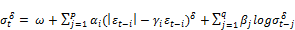(5)

where δ >0, i γ ≤1 for i =1, …, r, γi = 0 for all I > r, and r ≤ p If γ ≠ 0, the news impact is asymmetric.

The introduction and estimation of the power term in the APARCH model is an attempt to account for the true distribution underlying volatility . The idea behind the introduction of a power term arose from the fact that, The assumption of normality in modeling financial data, which restricts d to either 1 or 2, is often unrealistic due to significant skewness and kurtosis . Allowing d to take the form of a free parameter to be estimated removes this arbitrary restriction.

3. Recurrent Neural Networks

Neural networks can be classified into static and dynamic categories . Static networks have no feedback elements and contain no delays; the output is calculated directly from the input through feed forward connections. In dynamic networks, the output depends not only on the current input to the network, but also on the current or previous inputs, outputs, or states of the network. These dynamic networks may be recurrent networks with feedback connections or feed forward networks with imbedded tapped delay lines (or a hybrid of the two). For static networks, the standard back propagation algorithm  can be used to compute the gradient of the error function with respect to the network weights, which is needed for gradient-based training algorithms. For dynamic networks, a more complex dynamic gradient calculation must be performed. Although they can be trained using the same gradient-based algorithms that are used for static networks, the performance of the algorithms on dynamic network can be quite different and the gradient must be computed in a more complex way 

Dynamic networks are generally more powerful than static networks (although somewhat more difficult to train). Because dynamic networks have memory, they can be trained to learn sequential or time-varying patterns. This has applications in such disparate areas as prediction in financial markets , phase detection in power systems  and many more dynamic network applications in .

The Non-linear Auto-Regressive with Exogenous (NARX) inputs is a recurrent dynamic network, with feedback connections enclosing several layers of the network. The NARX model is based on the linear ARX model, which is commonly used in time-series modeling.

The defining equation for the NARX model is as follow: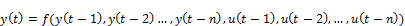(6)

Where the next value of the dependent output signal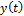is regressed on the previous values of the output signal and previous values of an independent (exogenous) input signals. A diagram showing the implementation of the NARX model using a feed forward neural network to approximate the function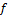in (6) is given in figure 1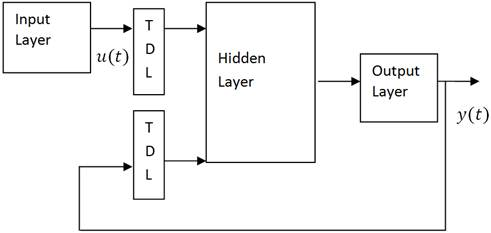Fig. 1. Implementation of NARX model.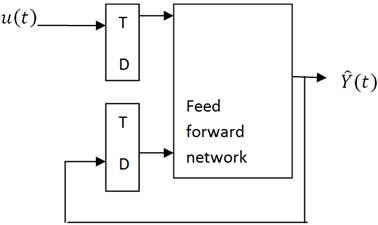Fig. 2. Parallel.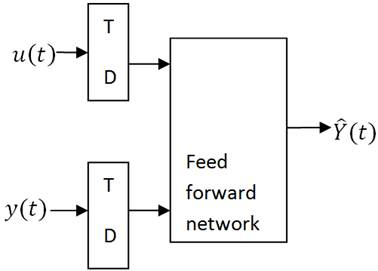Fig. 3. Series Parallel architectures.

Two different architectures have been proposed to train a NARX network . First is the parallel architecture as shown in figure 2, where the output of the neural network is fed back to the input of the feed forward neural network as part of the standard NARX architecture. In contrast, in the series-parallel architecture as shown in Figure 3, the true output of the volatility (not output of the identifier) is fed to the neural network model as it is available during the training. This has two advantages . The first is more accurate value presented as input to the neural network. The second advantage is the absence of a feedback loop in the network thereby enabling the use of static back propagation for training instead of the computationally expensive dynamic back propagation required for the parallel architecture. Also, assuming the output error tends to a small value asymptotically so that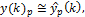the series parallel model may be replaced by a parallel model without serious consequences required.

4. GARCH-Neural Model

In this research, we proposed a hybrid model for forecasting volatility of the crude oil price in Nigeria. Initially, a preferred GARCH model is identified upon which the hybrid model is built. For this reason, optimum lags for GARCH model is estimated using AIC, and BIC indices.

The underlying concept for the hybrid model is that there are some explanatory factors other than historical prices that affect the future volatility crude oil price in the market. So this model is estimated with this number of variables that include squared prices, returns and it’s squared in addition to the historical prices of the crude oil. Now since the volatility estimated from preferred GARCH model is available during the training it is used as the target output to the network. Figure 4 and 5 shows the flowcharts schematic diagram of the system process respectively.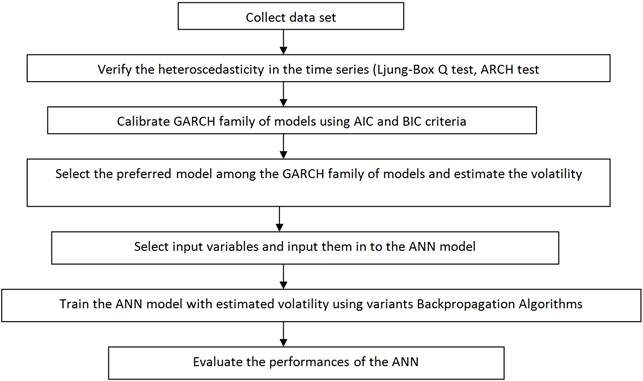Figure 4. Flowchart of training process of ANN.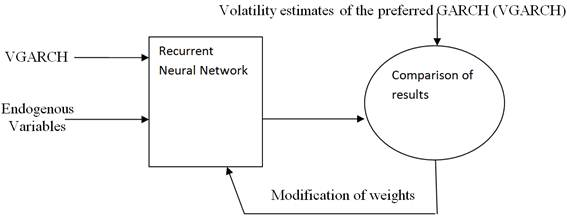Figure 5. Schematic representation of the system.

5. Training of the GARCH-Neural Model

Neural networks are fitted to the data by learning algorithms during a training process. In this research, supervised learning algorithms were employed. These learning algorithms are characterized by the usage of a given output that is compared to the predicted output and by the adaptation of all parameters according to this comparison. The parameters of a neural network are its weights. All weights are usually initialized with random values drawn from a standard normal distribution. During an iterative training process, the following steps are repeated:

1). The neural network calculates an output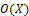for given inputs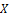and current weights. If the training process is not yet completed, the predicted output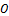will differ from the observed output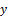.

2). An error function V, like the sum of squared errors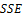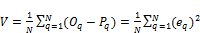(7)

Where, N is the size of the training dataset,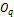and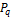are the target and predicted value of the output of the neural network when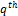input is presented andis the error (difference between the target and predicted value) for theinput. The performance index V in (7) is a function of weights and biases,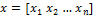and can be given by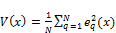(8)

The performance of the neural network can be improved by modifying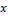till the desired level of the performance index,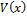is achieved. This is achieved by minimizingwith respect toand the gradient required for this is given by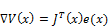(9)

Where,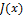is the Jacobian matrix given by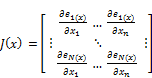(10)

and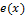is the error for all the inputs. The gradient in (8) is determined using back propagation algorithms, which involves performing computations backward through the network. The process stops if a pre-specified criterion is fulfilled, i.e. if the values of the gradient are smaller than a given threshold.

This gradient is then used by different algorithms to update the weights of the network. These algorithms differ in the way they use the gradient to update the weights of the network and are known as the variants of the back propagation algorithms.

Gradient descent algorithm with other variants is discussed below:

Gradient Descent algorithms (GD): The network weights and biases,is modified in a direction that reduces the performance function in (8) most rapidly i.e. the negative of the gradient of the performance function . The updated weights and biases in this algorithm are given by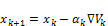(11)

Where,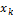is the vector of the current weights and biases,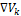is the current gradient of the performance function and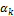is the learning rate.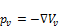(12)

The updated and weights and biases are then given by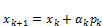(13)

Whereis the step size determined by the Levenberg-Marquardt algorithm . The next search direction that is conjugate to the previous search directions is determined by combining the new steepest descent direction with the previous search direction and is given by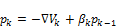(14)

The value of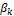is given in (Moller, 1993), by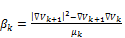(15)

Where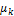is given by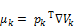(16)

Levenberg-Marquardt algorithm (LM): Since the performance index in (8) is sum of squares of non linear function, the numerical optimization techniques for non linear least squares can be used to minimize this cost function. The Levenberg-Marquardt algorithm, which is an approximation to the Newton’s method is said to be more efficient in comparison to other methods for convergence of the Backpropagation algorithm for training a moderate-sized feedforward neural network . As the cost function is a sum of squares of non linear function, the Hessian matrix required for updating the weights and biases need not be calculated and can be approximated as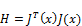(17)

The updated weights and biases are given by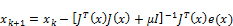(18)

Where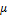is a scalar and I is the identity matrix.

Automated Bayesian Regularization (BR): Regularization as a mean of improving network generalization is used within the Levenberg-Marquardt algorithm. Regularization involves modification in the performance function. The performance function for this is the sum of the squares of the errors and it is modified to include a term that consists of the sum of squares of the network weights and biases. The modified performance function is given by(19)

Where SSE and SSW are given by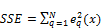(20)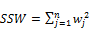(21)

Where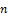is the total number of weights and biases,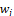in the network. The performance index in (19) forces the weights and biases to be small, which produces a smoother network response and avoids over fitting. The valuesand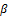are determined using Bayesian regularization in an automated manner  and .

6. Computational Results

The data for this research is obtained from central bank of Nigeria website www.cbn.gov.ng and has also been used in . It covers the monthly price of the crude oil from January, 1982 to February, 2016. Figure 6 represents the plot of both the crude oil price and its returns.

Table 1. Data description and preliminary statistics of the crude oil price reruns in Nigeria.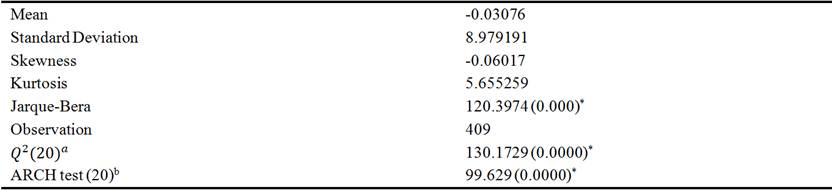ais the Ljung-Box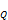test for the 15th order serial correlation of the squared returns

bEngle’s ARCH test also examines for autocorrelation of the squared returns.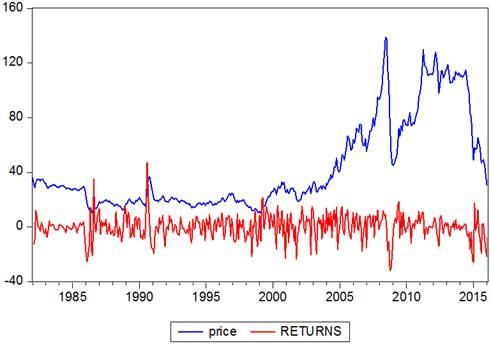Figure 6. Price and returns of the crude oil in Nigeria from 1982-2016.

With a brief glance at the table above, it can be seen that the mean of time series return in Nigerian crude oil price in the period under investigation is -0.030761 and its standard deviation is 8.79191. By comparing these two, it can be understood that this time series has experience a high level of volatility during this period. The Jarque-Bera test indicates non-normal distribution of this time series. Besides, the kurtosis statistics also indicate that the distribution of the mentioned time series is of fat tail. Observing the Liang-Box statistics (with twenty lags), can find, the null hypothesis about the lack of a serial correlation between the terms of the time series be rejected .

Now having confirmed from the table 1 above that the returns series of the crude oil price have ARCH effects, and then it is possible to estimate its conditional heteroscedasticity effects using GARCH models. Various combinations of (p, q) parameters ranging from (1, 1) to (3, 3) of GARCH models were calibrated on historical return data and best fitted ones were chosen from each group based on some certain performance measures as shown in table 2 .

Table 2. Information criteria for selecting best GARCH models.

 GARCH models AIC BIC GARCH (3, 3) -6.910500 -6.996954 EGARCH (3, 3) -6.961940 -7.036414 GJR (2, 2) -6.957762 -7.008816

By comparing information criteria related to different types of GARCH models, it can be easily found that the EGARCH (3, 3) model has the lowest Akaike and Schwarz information criteria, so, it is the best model for explaining the behavioral pattern of volatility in the crude price and is therefore chosen for the construction of the hybrid model.

The neural network proposed in section 4 was trained using training algorithms described in section 5. The dataset analyzed earlier in this section was used as training set. Table 2 summarizes the results of training the proposed network using the four training algorithms discussed above. Each entry in the table represents 100 different trials, with random weights taken for each trial to rule out the weight sensitivity of the performance of the different training algorithms. The network was trained in each case till the value of the performance index in (8) was 0.0001 or less.

Table 3. Descriptive statistics of different training algorithms.

 S/N Training Algorithm Average Time (s) Maximum Time (s) Minimum Tme (s) Standard Deviation 1 Gradient Descent Very slow in convergence 2 Scaled Conjugate Gradient Descent 145.3218 671.3451 98.3215 88.2381 3 Levenberg Marquardt 7.2137 10.4321 3.5437 2.5438 4 Bayesian Regularization 7.4532 11.0324 3.6718 2.1657

The Gradient Descent algorithm was generally shown very slow in convergence for the required value of the performance index. The average time required for training the network using the Levenberg- Marquardt algorithm was the smallest whereas, it took maximum time to train the network using the Scaled Conjugate Gradient Descent algorithm. The training algorithm employing Bayesian Regularization continuously modifies its performance function and hence, takes more time as compared to the Levenberg-Marquardt algorithm but this time is still far less than the Scaled Conjugate Gradient Descent method. From Table 2, it can be seen that the Levenberg-Marquardt algorithm is the fastest of all the training algorithms considered in this work for training a neural network to forecast the volatility. Since the training time required for different training algorithms have been compared, the conclusion drawn from the results for the offline training may also be extended to online training. Therefore, it can be assumed that similar trend of training time required by the different training algorithms will be exhibited during online training of the proposed model for continuous updating of the offline trained model.

Now the neural networks trained using the training algorithms listed in table 2 were tested. The datasets used for testing the networks were those points not included in the training set. Twenty set of data was considered. As the Gradient Descent algorithm was too low to converge for the desired value of the performance index, the neural networks that were trained using the rest of the three training algorithms listed in table 2 were tested using the datasets. The results for these are shown in the figures 7, 8 and 9 for Scale Conjugate Gradient, Levenberg-Marquardt and Bayesian Regularization respectively. The average absolute error is the least for the neural network trained using the LM method.

Table 4. Performance of trained neural networks on the datasets.

 S/No. Training Method Average Absolute Error for entire set Maximum Error Minimum Error 1 LM 0.001711 0.002901 0.000389 2 BR 0.04591 0.115341 0.0056 3 SCG 0.252393 0.506405 0.037923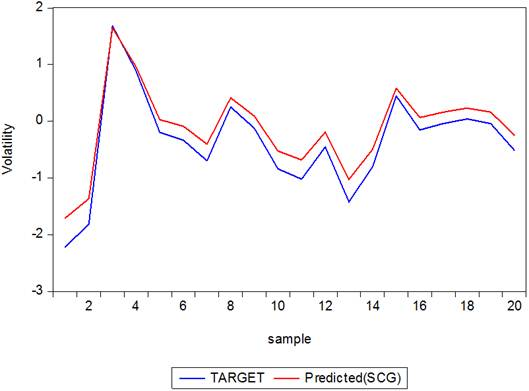Figure 7. Target and Predicted output of the GARCH-Neuron System using Scaled Conjugate Gradient.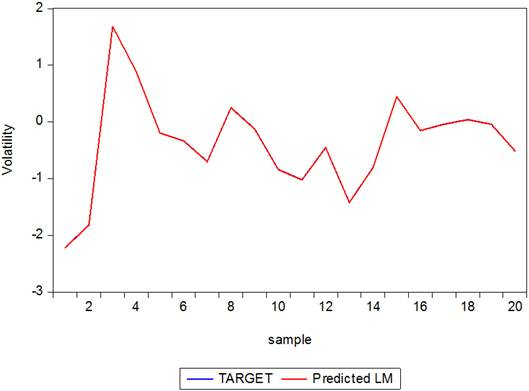Figure 8. Target and Predicted output of the GARCH-Neuron System using Levenberg-Marquardt.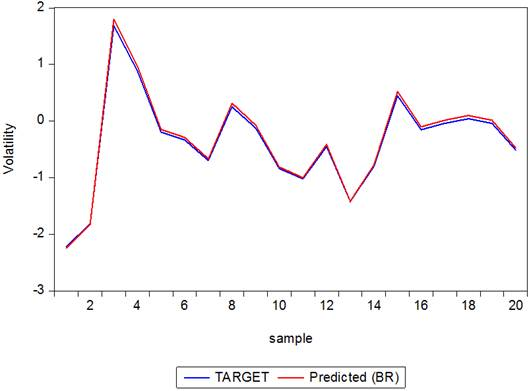Figure 9. Target and Predicted output of the GARCH-Neuron System using Bayesian Regularization.

7. Conclusion

A GARCH-Neural model has been proposed to forecast the volatility of the crude oil price in Nigeria. In the first place, A GARCH model was first identified upon which a hybrid model is built. EGARCH (3, 3) was identified to be preferred model for forecasting the volatility of crude oil price in Nigeria. Variants of the Backpropagation were used to train the proposed model. Investigations in to the training performance of the different algorithms show that the Levenberg-Marquardt (LM) algorithm is the fastest to converge. It was also established that LM algorithm gives most accurate predictions in comparisons to the target values of the volatility forecasted earlier by the preferred GARCH models.

References

1. Adamu A., (2015). The Impact of Global Fall in Oil Prices on the Nigerian Crude Oil Revenue and Its Prices, Proceedings of the Second Middle East Conference on Global Business, Economics, Finance and Banking Dubai-UAE, 22-24.
2. Mgbame C. O., Donwa P. A., Onyeokweni O. V., (2015). Impact of oil price volatility on Economic growth: Conceptual perspective, International Journal of Multidisciplinary Research and Development 9, 80-85.
3. Ogiri, I., H., Amadi, S., N., Uddin, M., M., & Dubon, P. (2013). Oil price and stock market performance in Nigeria: An empirical analysis. American Journal of Social and Management Sciences, 4(1), 20–41.
4. Oriakhi, D. E., &Osazee, I. D. (2013). Oil price volatility and its consequences on the growth of the Nigerian economy: An examination (1970-2010). Asian Economic and Financial Review, 3(5), 683-702.
5. Halpin, S. M. & Burch, R. F., (1997) "Applicability of neural networks to industrial and commercial power systems: a tutorial overview", IEEE Trans. Industry Applications, Vol. 33, No. 5, pp 1355-1361.
6. Hajizadeh E., Seifi A., Fazel Zarandi M. H., Turksen I. B., (2012). A hybrid modeling approach for forecasting the volatility of S&P 500 index return, Expert Systems with Applications, 39, 431-436.
7. Roman, J., and A. Jameel, (1996) "Backpropagation and recurrent neural networks in financial analysis of multiple stock market returns," Proceedings of the Twenty-Ninth Hawaii International Conference on System Sciences, Vol. 2, 1996, pp. 454–460.
8. Monfared S. A., and Enke D., (2014) "Volatility Forecasting using a Hybrid GJR-GARCH Neural Network model", Procedia Computer Science 36,246-253.
9. Donaldson R. G., and Kamstra M., (1997) "An artificial neural network-GARCH model for international stock return volatility", Journal of Empirical Finance, vol. 4, no. 1, pp. 17-46.
10. Mantri J. K., Gahan P., Nayak B. B., (2010) "Artificial Neural Networks - An Application to Stock Market Volatility", International Journal of Engineering Science and Technology, vol. 2, no. 5, pp. 1451-1460.
11. Jung-W. P., Venayagamoorthy, G. K., & Harley, R. G., (2005) "MLP/RBF neural networks based online global model identification of synchronous generator", IEEE Trans. Industrial Electronics, Vol. 52, No. 6, pp1685- 1695.
12. Venayagamoorthy, G. K., & Kalyani, R. P., (2005) "Two separate continually online-trained neurocontrollers for a unified power flow controller", IEEE Trans. Industry Applications, Vol. 41, No. 4, pp 906-916.
13. Mohamed, Y. A.-R., & El-Saadany, E. F., (2008) "Adaptive Discrete-Time Grid-Voltage Sensorless Interfacing Scheme for Grid-Connected DG-Inverters Based on Neural- Network Identification and Deadbeat Current Regulation", IEEE Trans. Power Electronics, Vol. 23, No. 1, pp308-321.
14. Tiwari, S., Naresh, R., & Jha, R., (2011) "Neural network predictive control of UPFC for improving transient stability performance of power system", Appl Soft Comput, Vol. 11, No. 8, pp4581-4590.
15. Engle, R. F., (1982). Autoregressive conditional heteroscedasticity with estimates of the variance of United Kingdom inflation, Econometrica, 50, 987-1007.
16. Bollerslev, T. (1986). Generalized autoregressive conditional heteroscedasticity. Journalof Econometrics, 31, 307–327.
17. Glosten, L. R. R. Jagannathan and D. Runkle. 1993. "On the Relation between the Expected Value and the Volatility of the Nominal Excess Return on Stocks." Journal of Finance. 48, 1779-1801.
18. Nelson, D. B. (1991). Conditional heteroskedasticity in asset returns: a new approach. Econo- metrica 59,347-370.
19. Longmore, R., and W. Robinson. 2004. "Modelling and Forecasting Exchange Rate Dynamics: An Application of Asymmetric Volatility Models". Bank of Jamaica. Working Paper WP2004/03.
20. Ding, Z. R. F. Engle and C. W. J. Granger. 1993. "Long Memory Properties of Stock Market Returns and a New Model". Journal of Empirical Finance. 1. 83–106.
21. Gupta M., Jin L., and Homma N., (2003) Static and Dynamic Neural Networks: From Fundamentals to Advanced Theory. New York: IEEE and Wiley.
22. Werbos P. J., The Roots of Backpropagation. New York: Wiley, 1994.
23. De Jesus, O. and M. T. Hagan, (2001a). Backpropagation through time for a general class of recurrent network. Proceedings of the international Joint Conference on Neural Networks, July 15-19, Washington, DC, USA., PP: 2638-2642.
24. Roman, J., and A. Jameel, "Backpropagation and recurrent neural networks in financial analysis of multiple stock market returns," Proceedings of the Twenty-Ninth Hawaii International Conference on System Sciences, Vol. 2, 1996, pp. 454–460.
25. Kamwa, I., R. Grondin, V. K. Sood, C. Gagnon, Van Thich Nguyen, and J. Mereb, "Recurrent neural networks for phasor detection and adaptive identification in power system control and protection," IEEE Transactions on Instrumentation and Measurement, Vol. 45, No. 2, 1996, pp. 657–664.
26. Medsker, L. R., and L. C. Jain(2000), Recurrent neural networks: design and applications, Boca Raton, FL: CRC Press.
27. Narendra, K. S., & Parthasarathy, K., (1990) "Identification and control of dynamical systems using neural networks", IEEE Trans. Neural Networks, Vol. 1, No. 1, pp4-27.
28. Hagan, M. T., Demuth, H. B., & Beale, M.H (1996) Neural Network Design, MA: PWS Publishing Boston.
29. Moller, M. F., (1993) "A scaled conjugate gradient algorithm for fast supervised learning", Neural Networks, Vol. 6, pp525-533.
30. Hagan, M. T., & Menhaj, M., (1994) "Training feed-forward networks with the Marquardt algorithm", IEEE Trans. Neural Networks, Vol. 5, No. 6, pp989-993.
31. Foresee, F. D. & Hagan, M. T., (1997) "Gauss-Newton approximation to Bayesian regularization", International Joint Conference on Neural Networks.
32. Mackay, D. J. C., (1992) "Bayesian interpolation", Neural Computation, Vol. 4, No. 3, pp415-447.
33. S. U. Gulumbe, S. Suleiman, B. K. Asare and M. Abubakar. Forecasting Volatility of Nigerian Crude Price Using Non-linear Auto-Regressive with Exogenous (NARX) Inputs Model, imperial journal of interdisciplinary Research (IJIR), Vol. 2, Issue 5, pp 434-442.

 Contents 1. 2. 3. 4. 5. 6. 7.
Article ToolsAbstractPDF(1676K)